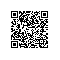# 【LeetCode】9. Palindrome Number

Determine whether an integer is a palindrome. Do this without extra space.

//c++
class Solution {
public:
bool isPalindrome(int x) {
int r=0,xc=x;
while(xc>0)
{
r*=10;
r+=xc%10;
xc/=10;
}
return r==x;
}
};使用钉钉扫一扫加入圈子
+ 订阅